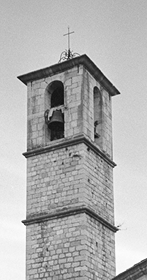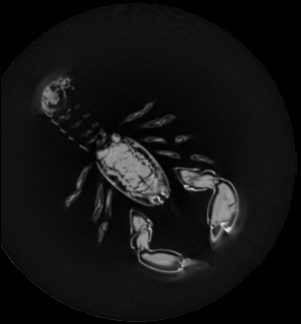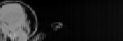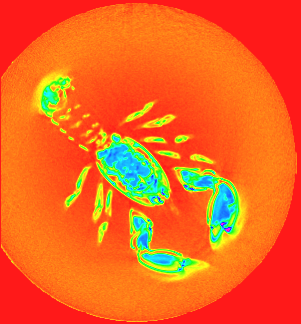DGtal  1.4.beta
Tutorial "Image -> ImageAdapter: some simple image manipulations"

Author(s) of this documentation:
Bertrand Kerautret

# Preliminary

The GenericReader and GenericWriter class allow you to import/export different image formats depending of the optional DGtal dependancies (ITK).

Todo:
Exercise Construct a simple file format converter starting from this file template:
#include "DGtal/base/Common.h"
#include "DGtal/images/ImageContainerBySTLVector.h"
#include "DGtal/io/writers/GenericWriter.h"
#include "DGtal/helpers/StdDefs.h"
#include <boost/program_options/options_description.hpp>
#include <boost/program_options/parsers.hpp>
#include <boost/program_options/variables_map.hpp>
using namespace std;
using namespace DGtal;
using namespace Z3i;
namespace po = boost::program_options;
int main( int argc, char** argv )
{
typedef ImageContainerBySTLVector<Z2i::Domain, unsigned char> Image2D;
// parse command line ----------------------------------------------
po::options_description general_opt ( "Allowed options are: " );
( "help,h", "display this message." )
( "input,i", po::value<std::string>(), "Input vol file." )
( "output,o", po::value<string>(),"Output OFF filename." );
bool parseOK=true;
po::variables_map vm;
try{
po::store(po::parse_command_line(argc, argv, general_opt), vm);
}catch(const std::exception& ex){
parseOK=false;
trace.info()<< "Error checking program options: "<< ex.what()<< endl;
}
po::notify ( vm );
if (!parseOK || vm.count ( "help" ) ||argc<=1 || !vm.count("input")||! vm.count("output"))
{
trace.info() << "exercise ImageIO."<<std::endl
<< std::endl << "Basic usage: "<<std::endl
<< "\tvol2off --input <volFileName> --o <OffOutputFileName> "<<std::endl
<< general_opt << "\n";
return 0;
}
return 0;
}
std::ostream & info()
DGtal is the top-level namespace which contains all DGtal functions and types.
Trace trace
Definition: Common.h:153
int main(int argc, char **argv)

Complements:

• Test the same reading/export on 3D volume (vol pgm3D format).

# Basic Image manipulations

The aim of this tutorial is to propose some basic image manipulations in particular by exploiting the image adapter classes (ImageAdapter) with different functors.

## Image cropping

The ImageAdapter and ConstImageAdapter can be very efficient to define for instance a cropped image (see documentation given in the Images module). To persform a simple crop you can apply the following steps:

• Load the source image to be cropped.
• Define type associated to the ConstImageAdapter.
• Define a sub domain and construct the cropped image.
• Export the result.

If you use image of DGtal/examples/samples/church.pgm with the given domain, you should obtain the following image:Image cropped to the domain defined by points (170,466) and (316, 745).

Complements:

• Add options to define the input/output files and region bounds.
• Apply the same cropping to 3D images.

## 2D slice image extraction from 3D volume

In the same spirit than previous example, you can also extract a 2D slice image from a 3D volume image.

To apply the extraction you can follow these steps:

• Construct a ConstImageAdapter with the following types:
• type of the source Image (Image3D).
• the domain type of the resulting image (Image2D::Domain).
• the functor taking in input 2D data and return 3D points ( DGtal::functors::Projector<Z3i::Space>) to access to source image data.
• the type of value of the 3D image (Image3D::Value)
• the functor type to be applied on the source image value during the reading process.
• Construct the domain of the resulting image.
• Construct the functor to embed the 2D point into the 3D volume (by using the Projector class returning 3D points from 2D coordinates).

If you have used the vol file: DGtal/examples/samples/lobster.vol and a slice number, you should obtain the following image:Result of slice image number 20 on Z axis.
Todo:
Complementary exercise:
• Apply the same slice extraction on axis X and Y intersecting the center point of the 3D Image.

## 2D Image extraction from 3D volume

The functor Point2DEmbedderIn3D defined in the BasicPointFunctors class allows to embed a point of an 2D domain into a 3D one. By this way you can extract a 2D image from a 3D volume just by using this functor as pointFunctor in the ConstImageAdapter.

To construct a Point2DEmbedderIn3D you have to give the domain of the 3d image, the origin point with an upper point on axis1 and on axis2.

Todo:
Exercise: Construct a program able to extract an 2D image from a given volume and 3 3D points. For instance you can to extract the 2D image defined by the points:
• Origin (120, 150, 10)
• Upper point1 (220, 220, 10)
• Upper point2 (120, 150, 50) You should obtain the following 2D image:Result of slice image number 20 on Z axis.

## Create image with color Functor

By using the HueShadeColorMap as functor in the exportPPM method (of PPMWriter), transform a grayscale image into color. For instance with the previous image you should obtain: